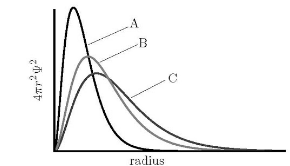# Problem: The graph shows the radial distribution plots for the 1s wavefunction for H, He, and He+. Which plot is the 1s wavefunction for the He + ion? 1. A 2. B 3. There is no way to know 4. C

###### FREE Expert Solution
89% (391 ratings)
###### Problem Details

The graph shows the radial distribution plots for the 1s wavefunction for H, He, and He+.

Which plot is the 1s wavefunction for the He + ion?

1. A

2. B

3. There is no way to know

4. CWhat scientific concept do you need to know in order to solve this problem?

Our tutors have indicated that to solve this problem you will need to apply the Wave Function concept. If you need more Wave Function practice, you can also practice Wave Function practice problems.

What is the difficulty of this problem?

Our tutors rated the difficulty ofThe graph shows the radial distribution plots for the 1s wav...as medium difficulty.

How long does this problem take to solve?

Our expert Chemistry tutor, Jules took 3 minutes and 53 seconds to solve this problem. You can follow their steps in the video explanation above.

What professor is this problem relevant for?

Based on our data, we think this problem is relevant for Professor Lee's class at CORNELL.